$$\newcommand{\id}{\mathrm{id}}$$ $$\newcommand{\Span}{\mathrm{span}}$$ $$\newcommand{\kernel}{\mathrm{null}\,}$$ $$\newcommand{\range}{\mathrm{range}\,}$$ $$\newcommand{\RealPart}{\mathrm{Re}}$$ $$\newcommand{\ImaginaryPart}{\mathrm{Im}}$$ $$\newcommand{\Argument}{\mathrm{Arg}}$$ $$\newcommand{\norm}{\| #1 \|}$$ $$\newcommand{\inner}{\langle #1, #2 \rangle}$$ $$\newcommand{\Span}{\mathrm{span}}$$

# 3.4: Triangles, Rectangles, and the Pythagorean Theorem

$$\newcommand{\vecs}{\overset { \rightharpoonup} {\mathbf{#1}} }$$ $$\newcommand{\vecd}{\overset{-\!-\!\rightharpoonup}{\vphantom{a}\smash {#1}}}$$$$\newcommand{\id}{\mathrm{id}}$$ $$\newcommand{\Span}{\mathrm{span}}$$ $$\newcommand{\kernel}{\mathrm{null}\,}$$ $$\newcommand{\range}{\mathrm{range}\,}$$ $$\newcommand{\RealPart}{\mathrm{Re}}$$ $$\newcommand{\ImaginaryPart}{\mathrm{Im}}$$ $$\newcommand{\Argument}{\mathrm{Arg}}$$ $$\newcommand{\norm}{\| #1 \|}$$ $$\newcommand{\inner}{\langle #1, #2 \rangle}$$ $$\newcommand{\Span}{\mathrm{span}}$$ $$\newcommand{\id}{\mathrm{id}}$$ $$\newcommand{\Span}{\mathrm{span}}$$ $$\newcommand{\kernel}{\mathrm{null}\,}$$ $$\newcommand{\range}{\mathrm{range}\,}$$ $$\newcommand{\RealPart}{\mathrm{Re}}$$ $$\newcommand{\ImaginaryPart}{\mathrm{Im}}$$ $$\newcommand{\Argument}{\mathrm{Arg}}$$ $$\newcommand{\norm}{\| #1 \|}$$ $$\newcommand{\inner}{\langle #1, #2 \rangle}$$ $$\newcommand{\Span}{\mathrm{span}}$$

Learning Objectives

By the end of this section, you will be able to:

• Solve applications using properties of triangles
• Use the Pythagorean Theorem
• Solve applications using rectangle properties

Before you get started, take this readiness quiz.

1. Simplify: $$12(6h)$$.
If you missed this problem, review Exercise 1.10.1.
2. The length of a rectangle is three less than the width. Let w represent the width. Write an expression for the length of the rectangle.
If you missed this problem, review Exercise 1.3.43.
3. Solve: $$A=\frac{1}{2}bh$$ for b when A=260 and h=52.
If you missed this problem, review Exercise 2.6.10.
4. Simplify: $$\sqrt{144}$$.
If you missed this problem, review Exercise 1.9.10.

## Solve Applications Using Properties of Triangles

In this section we will use some common geometry formulas. We will adapt our problem-solving strategy so that we can solve geometry applications. The geometry formula will name the variables and give us the equation to solve. In addition, since these applications will all involve shapes of some sort, most people find it helpful to draw a figure and label it with the given information. We will include this in the first step of the problem solving strategy for geometry applications.

SOLVE GEOMETRY APPLICATIONS

1. Read the problem and make sure all the words and ideas are understood. Draw the figure and label it with the given information.
2. Identify what we are looking for.
3. Label what we are looking for by choosing a variable to represent it.
4. Translate into an equation by writing the appropriate formula or model for the situation. Substitute in the given information.
5. Solve the equation using good algebra techniques.
6. Check the answer by substituting it back into the equation solved in step 5 and by making sure it makes sense in the context of the problem.
7. Answer the question with a complete sentence.

We will start geometry applications by looking at the properties of triangles. Let’s review some basic facts about triangles. Triangles have three sides and three interior angles. Usually each side is labeled with a lowercase letter to match the uppercase letter of the opposite vertex.

The plural of the word vertex is vertices. All triangles have three vertices. Triangles are named by their vertices: The triangle in Figure $$\PageIndex{1}$$ is called $$\triangle{ABC}$$.Figure $$\PageIndex{1}$$: Triangle ABC has vertices $$A$$, $$B$$, and $$C$$. The lengths of the sides are $$a$$, $$b$$, and $$c$$.

The three angles of a triangle are related in a special way. The sum of their measures is $$180^{\circ}$$. Note that we read $$m\angle{A}$$ as “the measure of angle A.” So in $$\triangle{ABC}$$ in Figure $$\PageIndex{1}$$.

$m \angle A+m \angle B+m \angle C=180^{\circ} \nonumber$

Because the perimeter of a figure is the length of its boundary, the perimeter of $$\triangle{ABC}$$ is the sum of the lengths of its three sides.

$P = a + b + c \nonumber$

To find the area of a triangle, we need to know its base and height. The height is a line that connects the base to the opposite vertex and makes a $$90^\circ$$ angle with the base. We will draw $$\triangle{ABC}$$ again, and now show the height, $$h$$. See Figure $$\PageIndex{2}$$.Figure $$\PageIndex{2}$$: The formula for the area of $$\triangle{ABC}$$ is $$A = \frac{1}{2}bh$$ where b is the base and h is the height.

TRIANGLE PROPERTIESFor $$\triangle{ABC}$$

Angle measures:

$m \angle A+m \angle B+m \angle C=180^{\circ}$

• The sum of the measures of the angles of a triangle is 180°.

Perimeter:

$P = a + b + c$

• The perimeter is the sum of the lengths of the sides of the triangle.

Area:

$$A = \frac{1}{2}bh, b = \text{ base }, h = \text{ height }$$

• The area of a triangle is one-half the base times the height.

Example $$\PageIndex{1}$$

The measures of two angles of a triangle are 55 and 82 degrees. Find the measure of the third angle.

Solution

 Step 1. Read the problem. Draw the figure and label it with the given information.Step 2. Identify what you are looking for. the measure of the third angle in a triangle Step 3. Name. Choose a variable to represent it. Let $$x=$$ the measure of the angle. Step 4. Translate. Write the appropriate formula and substitute. $$m \angle A+m \angle B+m \angle C=180^{\circ}$$ Step 5. Solve the equation. $$\begin{array} {rll} {55 + 82 + x} &{=} &{180} \\ {137 + x} &{=} &{180} \\ {x} &{=} &{43} \end{array}$$ Step 6. Check. $$\begin{array} {rll} {55 + 82 + 43} &{\stackrel{?}{=}} &{180} \\ {180} &{=} &{180\checkmark} \end{array}$$ Step 7. Answer the question. The measure of the third angle is 43 degrees.

Try It $$\PageIndex{1}$$

The measures of two angles of a triangle are 31 and 128 degrees. Find the measure of the third angle.

21 degrees

Try It $$\PageIndex{2}$$

The measures of two angles of a triangle are 49 and 75 degrees. Find the measure of the third angle.

56 degrees

Example $$\PageIndex{2}$$

The perimeter of a triangular garden is 24 feet. The lengths of two sides are four feet and nine feet. How long is the third side?

Solution

 Step 1. Read the problem. Draw the figure and label it with the given information.Step 2. Identify what you are looking for. length of the third side of a triangle Step 3. Name. Choose a variable to represent it. Let $$c=$$ the third side. Step 4. Translate. Write the appropriate formula and substitute.Substitute in the given information.Step 5. Solve the equation.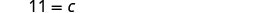Step 6. Check. $$\begin{array} {rll} {P} &{=} &{a + b +c} \\ {24} &{\stackrel{?}{=}} &{4 + 9+11} \\ {24} &{=} &{24\checkmark} \end{array}$$ Step 7. Answer the question. The third side is 11 feet long.

Try It $$\PageIndex{3}$$

The perimeter of a triangular garden is 48 feet. The lengths of two sides are 18 feet and 22 feet. How long is the third side?

8 feet

Try It $$\PageIndex{4}$$

The lengths of two sides of a triangular window are seven feet and five feet. The perimeter is 18 feet. How long is the third side?

6 feet

Example $$\PageIndex{3}$$

The area of a triangular church window is 90 square meters. The base of the window is 15 meters. What is the window’s height?

Solution

 Step 1. Read the problem. Draw the figure and label it with the given information.$$\text{ Area } = 90m^{2}$$ Step 2. Identify what you are looking for. height of a triangle Step 3. Name. Choose a variable to represent it. Let $$h=$$ the height. Step 4. Translate. Write the appropriate formula.Substitute in the given information.Step 5. Solve the equation. $$90 = \dfrac{15}{2}h$$ $$12 = h$$ Step 6. Check. $$\begin{array} {rll} {A} &{=} &{\frac{1}{2}bh} \\ {90} &{\stackrel{?}{=}} &{\frac{1}{2}\cdot 15\cdot 12} \\ {90} &{=} &{90\checkmark} \end{array}$$ Step 7. Answer the question. The height of the triangle is 12 meters.

Try It $$\PageIndex{5}$$

The area of a triangular painting is 126 square inches. The base is 18 inches. What is the height?

14 inches

Try It $$\PageIndex{6}$$

A triangular tent door has area 15 square feet. The height is five feet. What is the base?

6 feet

The triangle properties we used so far apply to all triangles. Now we will look at one specific type of triangle—a right triangle. A right triangle has one 90° angle, which we usually mark with a small square in the corner.Figure $$\PageIndex{3}$$

Definition: RIGHT TRIANGLE

A right triangle has one 90° angle, which is often marked with a square at the vertex.

Example $$\PageIndex{4}$$

One angle of a right triangle measures 28°. What is the measure of the third angle?

Solution

 Step 1. Read the problem. Draw the figure and label it with the given information.Step 2. Identify what you are looking for. the measure of an angle Step 3. Name. Choose a variable to represent it. Let $$x=$$ the measure of an angle. Step 4. Translate. $$m\angle{A} + m\angle{B} + m\angle{C} = 180$$ Write the appropriate formula and substitute. $$x+90+28=180$$ Step 5. Solve the equation. $$x=62$$ Step 6. Check. $$\begin{array} {rll} {180} &{\stackrel{?}{=}} &{90+28+62} \\ {180} &{=} &{180\checkmark} \end{array}$$ Step 7. Answer the question. The measure of the third angle is 62°.

Try It $$\PageIndex{7}$$

One angle of a right triangle measures 56°. What is the measure of the other small angle?

34°

Try It $$\PageIndex{8}$$

One angle of a right triangle measures 45°. What is the measure of the other small angle?

45°

In the examples we have seen so far, we could draw a figure and label it directly after reading the problem. In the next example, we will have to define one angle in terms of another. We will wait to draw the figure until we write expressions for all the angles we are looking for.

Example $$\PageIndex{5}$$

The measure of one angle of a right triangle is 20 degrees more than the measure of the smallest angle. Find the measures of all three angles.

Solution

 Step 1. Read the problem. Step 2. Identify what you are looking for. the measures of all three angles Step 3. Name. Choose a variable to represent it. Let $$a=1^{st}$$ angle. $$a+20=2^{nd}$$ angle $$90=3^{rd}$$ angle (the right angle) Draw the figure and label it with the given informationStep 4. TranslateWrite the appropriate formula. Substitute into the formula. $$a + (a + 20) + 90 = 180$$ Step 5. Solve the equation. \begin{align*} 2a + 110 &= 180 \3pt] 2a &= 70 \\[3pt] a &= 35 \text{ first angle}\\[3pt] a &+ 20 \text{ second angle}\\[3pt] {\color{red}{35}} &+ 20 = 55 \end{align*} And the third angle is 90. Step 6. Check. $$\begin{array} {rll} {35 + 55 + 90} &{\stackrel{?}{=}} &{180} \\ {180} &{=} &{180\checkmark} \end{array}$$ Step 7. Answer the question. The three angles measure 35°, 55°, and 90°. Try It $$\PageIndex{9}$$ The measure of one angle of a right triangle is 50° more than the measure of the smallest angle. Find the measures of all three angles. Answer 20°,70°,90° Try It $$\PageIndex{10}$$ The measure of one angle of a right triangle is 30° more than the measure of the smallest angle. Find the measures of all three angles. Answer 30°,60°,90° ## Use the Pythagorean Theorem We have learned how the measures of the angles of a triangle relate to each other. Now, we will learn how the lengths of the sides relate to each other. An important property that describes the relationship among the lengths of the three sides of a right triangle is called the Pythagorean Theorem. This theorem has been used around the world since ancient times. It is named after the Greek philosopher and mathematician, Pythagoras, who lived around 500 BC. Before we state the Pythagorean Theorem, we need to introduce some terms for the sides of a triangle. Remember that a right triangle has a 90° angle, marked with a small square in the corner. The side of the triangle opposite the 90°90° angle is called the hypotenuse and each of the other sides are called legs.Figure $$\PageIndex{4}$$ The Pythagorean Theorem tells how the lengths of the three sides of a right triangle relate to each other. It states that in any right triangle, the sum of the squares of the lengths of the two legs equals the square of the length of the hypotenuse. In symbols we say: in any right triangle, $$a^{2}+b^{2}=c^{2}$$, where a and b are the lengths of the legs and cc is the length of the hypotenuse. Writing the formula in every exercise and saying it aloud as you write it, may help you remember the Pythagorean Theorem. THE PYTHAGOREAN THEOREM In any right triangle, where $$a$$ and $$b$$ are the lengths of the legs, $$c$$ is the length of the hypotenuse.Then \[a^{2}+b^{2}=c^{2} \label{Ptheorem}

To solve exercises that use the Pythagorean Theorem (Equation \ref{Ptheorem}), we will need to find square roots. We have used the notation $$\sqrt{m}$$ and the definition:

If $$m = n^{2}$$, then $$\sqrt{m} = n$$, for $$n\geq 0$$.

For example, we found that $$\sqrt{25}$$ is 5 because $$25=5^{2}$$.

Because the Pythagorean Theorem contains variables that are squared, to solve for the length of a side in a right triangle, we will have to use square roots.

Example $$\PageIndex{6}$$

Use the Pythagorean Theorem to find the length of the hypotenuse shown below.Solution

 Step 1. Read the problem. Step 2. Identify what you are looking for. the length of the hypotenuse of the triangle Step 3. Name. Choose a variable to represent it. Label side c on the figure. Let c = the length of the hypotenuse.Step 4. Translate. Write the appropriate formula. $$a^{2} + b^{2} = c^{2}$$ Substitute. $$3^{2}+4^{2}=c^{2}$$ Step 5. Solve the equation. $$9+16=c^{2}$$ Simplify. $$25=c^{2}$$ Use the definition of square root. $$\sqrt{25} = c$$ Simplify. $$5=c$$ Step 6. Check.Step 7. Answer the question. The length of the hypotenuse is 5.

Try It $$\PageIndex{11}$$

Use the Pythagorean Theorem to find the length of the hypotenuse in the triangle shown below.c=10

Try It $$\PageIndex{12}$$

Use the Pythagorean Theorem to find the length of the hypotenuse in the triangle shown below.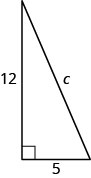c=13

Example $$\PageIndex{7}$$

Use the Pythagorean Theorem to find the length of the leg shown below.Solution

 Step 1. Read the problem. Step 2. Identify what you are looking for. the length of the leg of the triangle Step 3. Name. Choose a variable to represent it. Let $$b=$$ the leg of the triangle. Label side $$b$$.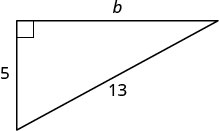Step 4. Translate Write the appropriate formula. $$a^{2} + b^{2} = c^{2}$$ Substitute. $$5^{2}+b^{2}=13^{2}$$ Step 5. Solve the equation. $$25+b^{2}=169$$ Isolate the variable term. $$b^{2}=144$$ Use the definition of square root. $$b = \sqrt{144}$$ Simplify. $$b=12$$ Step 6. Check.Step 7. Answer the question. The length of the leg is 12.

Try It $$\PageIndex{13}$$

Use the Pythagorean Theorem to find the length of the leg in the triangle shown below.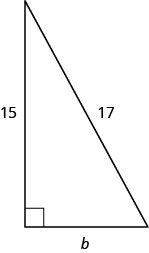8

Try It $$\PageIndex{14}$$

Use the Pythagorean Theorem to find the length of the leg in the triangle shown below.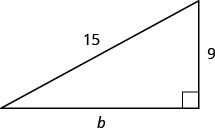12

Example $$\PageIndex{8}$$Kelvin is building a gazebo and wants to brace each corner by placing a 10″ piece of wood diagonally as shown above.

If he fastens the wood so that the ends of the brace are the same distance from the corner, what is the length of the legs of the right triangle formed? Approximate to the nearest tenth of an inch.

Solution

$$\begin{array} {ll} {\textbf{Step 1. }\text{Read the problem.}} &{} \\\\ {\textbf{Step 2. }\text{Identify what we are looking for.}} &{\text{the distance from the corner that the}} \\ {} &{\text{bracket should be attached}} \\ \\{\textbf{Step 3. }\text{Name. Choose a variable to represent it.}} &{\text{Let x = distance from the corner.}} \\ {\textbf{Step 4.} \text{Translate}} &{} \\ {\text{Write the appropriate formula and substitute.}} &{a^{2} + b^{2} = c^{2}} \\ {} &{x^{2} + x^{2} = 10^{2}} \\ \\ {\textbf{Step 5. Solve the equation.}} &{} \\ {} &{2x^{2} = 100} \\ {\text{Isolate the variable.}} &{x^{2} = 50} \\ {\text{Simplify. Approximate to the nearest tenth.}} &{x \approx 7.1}\\\\ {\textbf{Step 6. }\text{Check.}} &{}\\ {a^{2} + b^{2} = c^{2}} &{} \\ {(7.1)^{2} + (7.1)^{2} \approx 10^{2} \text{ Yes.}} &{} \\\\ {\textbf{Step 7. Answer the question.}} &{\text{Kelven should fasten each piece of}} \\ {} &{\text{wood approximately 7.1'' from the corner.}} \end{array}$$

Try It $$\PageIndex{15}$$

John puts the base of a 13-foot ladder five feet from the wall of his house as shown below. How far up the wall does the ladder reach?12 feet

Try It $$\PageIndex{16}$$

Randy wants to attach a 17 foot string of lights to the top of the 15 foot mast of his sailboat, as shown below. How far from the base of the mast should he attach the end of the light string?8 feet

## Solve Applications Using Rectangle Properties

You may already be familiar with the properties of rectangles. Rectangles have four sides and four right (90°) angles. The opposite sides of a rectangle are the same length. We refer to one side of the rectangle as the length, $$L$$, and its adjacent side as the width, $$W$$.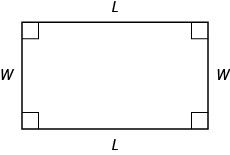The distance around this rectangle is $$L+W+L+W$$, or $$2L+2W$$. This is the perimeter, $$P$$, of the rectangle.

$P=2L+2W$

What about the area of a rectangle? Imagine a rectangular rug that is 2-feet long by 3-feet wide. Its area is 6 square feet. There are six squares in the figure.$\begin{array} {l} {A=6} \\ {A=2\cdot3} \\ {A=L\cdot W} \end{array}$

The area is the length times the width. The formula for the area of a rectangle is

$A=LW.$

PROPERTIES OF RECTANGLES

Rectangles have four sides and four right (90°) angles.

The lengths of opposite sides are equal.

The perimeter of a rectangle is the sum of twice the length and twice the width.

$P=2L+2W$

The area of a rectangle is the product of the length and the width.

$A=L·W$

Example $$\PageIndex{9}$$

The length of a rectangle is 32 meters and the width is 20 meters. What is the perimeter?

Solution

 Step 1. Read the problem. Draw the figure and label it with the given information.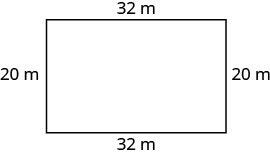Step 2. Identify what you are looking for. the perimeter of a rectangle Step 3. Name. Choose a variable to represent it. Let $$P=$$ the perimeter. Step 4. Translate. Write the appropriate formula.Substitute.Step 5. Solve the equation. $$P = 64 + 40$$ $$P = 104$$ Step 6. Check. $$\begin{array} {rcl} {P} &{\stackrel{?}{=}} &{104} \\ {20+32+20+32} &{\stackrel{?}{=}} &{104} \\ {104} &{=} &{104\checkmark} \end{array}$$ Step 7. Answer the question. The perimeter of the rectangle is 104 meters.

Try It $$\PageIndex{17}$$

The length of a rectangle is 120 yards and the width is 50 yards. What is the perimeter?

340 yards

Try It $$\PageIndex{18}$$

The length of a rectangle is 62 feet and the width is 48 feet. What is the perimeter?

220 feet

Example $$\PageIndex{10}$$

The area of a rectangular room is 168 square feet. The length is 14 feet. What is the width?

Solution

 Step 1. Read the problem. Draw the figure and label it with the given information.Step 2. Identify what you are looking for. the width of a rectangular room Step 3. Name. Choose a variable to represent it. Let $$W=$$ the width. Step 4. Translate. Write the appropriate formula. $$A=LW$$ Substitute. $$168 = 14W$$ Step 5. Solve the equation. $$\frac{168}{14} = \frac{14W}{14}$$ $$12 = W$$ Step 6. Check.$$\begin{array} {rcl} {A} &{=} &{LW} \\ {168} &{\stackrel{?}{=}} &{14\cdot 12} \\ {168} &{=} &{168\checkmark} \end{array}$$ Step 7. Answer the question. The width of the room is 12 feet.

Try It $$\PageIndex{19}$$

The area of a rectangle is 598 square feet. The length is 23 feet. What is the width?

26 feet

Try It $$\PageIndex{20}$$

The width of a rectangle is 21 meters. The area is 609 square meters. What is the length?

29 meters

Example $$\PageIndex{11}$$

Find the length of a rectangle with perimeter 50 inches and width 10 inches.

Solution

 Step 1. Read the problem. Draw the figure and label it with the given information.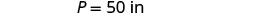Step 2. Identify what you are looking for. the length of the rectangle Step 3. Name. Choose a variable to represent it. Let $$L=$$ the length. Step 4. Translate. Write the appropriate formula. $$P = 2L + 2W$$ Substitute. $$50 = 2L + 2(10)$$ Step 5. Solve the equation.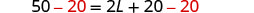Step 6. Check.$$\begin{array} {rcl} {P} &{=} &{50} \\ {15+10+15+10} &{\stackrel{?}{=}} &{50} \\ {50} &{=} &{50\checkmark} \end{array}$$ Step 7. Answer the question. The length is 15 inches.

Try It $$\PageIndex{21}$$

Find the length of a rectangle with: perimeter 80 and width 25.

15

Try It $$\PageIndex{22}$$

Find the length of a rectangle with: perimeter 30 and width 6.

9

We have solved problems where either the length or width was given, along with the perimeter or area; now we will learn how to solve problems in which the width is defined in terms of the length. We will wait to draw the figure until we write an expression for the width so that we can label one side with that expression.

Example $$\PageIndex{12}$$

The width of a rectangle is two feet less than the length. The perimeter is 52 feet. Find the length and width.

Solution

 Step 1. Read the problem. Step 2. Identify what you are looking for. the length and width of a rectangle Step 3. Name. Choose a variable to represent it. Since the width is defined in terms of the length, we let $$L=$$ length. The width is two feet less than the length, so we let $$L-2$$ width.$$P=52$$ ft Step 4. Translate. Write the appropriate formula. The formula for the perimeter of a rectangle relates all the information. $$P=2L+2W$$ Substitute in the given information. $$52=2L+2(L−2)$$ Step 5. Solve the equation. $$52=2L+2L−4$$ Combine like terms. $$52=4L−4$$ Add 4 to each side. $$56 = 4L$$ Divide by 4. $$\frac{56}{4} = \frac{4L}{4}$$ $$14=L$$ The length is 14 feet. Now we need to find the width. The width is $$L−2$$.The width is 12 feet. Step 6. Check. Since $$14+12+14+12=52$$, this works!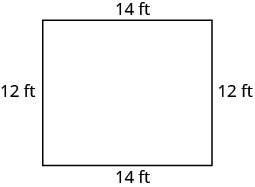Step 7. Answer the question. The length is 14 feet and the width is 12 feet.

Try It $$\PageIndex{23}$$

The width of a rectangle is seven meters less than the length. The perimeter is 58 meters. Find the length and width.

18 meters, 11 meters

Try It $$\PageIndex{24}$$

The length of a rectangle is eight feet more than the width. The perimeter is 60 feet. Find the length and width.

19 feet, 11 feet

Example $$\PageIndex{13}$$

The length of a rectangle is four centimeters more than twice the width. The perimeter is 32 centimeters. Find the length and width.

Solution

 Step 1. Read the problem. Step 2. Identify what you are looking for. the length and the width Step 3. Name. Choose a variable to represent the width.The length is four more than twice the width.Step 4. Translate Write the appropriate formula. $$\quad P=2L+2W$$ Substitute in the given information.Step 5. Solve the equation.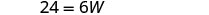12 The length is 12 cm. Step 6. Check.$$\begin{array} {rcl} {P} &{=} &{2L + 2W} \\ {32} &{\stackrel{?}{=}} &{2\cdot 12 + 2\cdot 4} \\ {32} &{=} &{32\checkmark} \end{array}$$ Step 7. Answer the question. The length is 12 cm and the width is 4 cm.

Try It $$\PageIndex{25}$$

The length of a rectangle is eight more than twice the width. The perimeter is 64. Find the length and width.

24, 8

Try It $$\PageIndex{26}$$

The width of a rectangle is six less than twice the length. The perimeter is 18. Find the length and width.

5, 4

Example $$\PageIndex{14}$$

The perimeter of a rectangular swimming pool is 150 feet. The length is 15 feet more than the width. Find the length and width.

Solution

 Step 1. Read the problem. Draw the figure and label it with the given information.$$P=150$$ ft Step 2. Identify what you are looking for. the length and the width of the pool Step 3. Name. Choose a variable to represent the width. The length is 15 feet more than the width.Step 4. Translate Write the appropriate formula. $$\quad P=2L+2W$$ Substitute.Step 5. Solve the equation.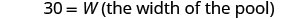Step 6. Check. $$\begin{array} {rcl} {P} &{=} &{2L + 2W} \\ {150} &{\stackrel{?}{=}} &{2(45) + 2(30)} \\ {150} &{=} &{150\checkmark} \end{array}$$ Step 7. Answer the question. The length of the pool is 45 feet and the width is 30 feet.

Try It $$\PageIndex{27}$$

The perimeter of a rectangular swimming pool is 200 feet. The length is 40 feet more than the width. Find the length and width.

70 feet, 30 feet

Try It $$\PageIndex{28}$$

The length of a rectangular garden is 30 yards more than the width. The perimeter is 300 yards. Find the length and width.

90 yards, 60 yards

## Key Concepts

• Problem-Solving Strategy for Geometry Applications
1. Read the problem and make all the words and ideas are understood. Draw the figure and label it with the given information.
2. Identify what we are looking for.
3. Name what we are looking for by choosing a variable to represent it.
4. Translate into an equation by writing the appropriate formula or model for the situation. Substitute in the given information.
5. Solve the equation using good algebra techniques.
6. Check the answer in the problem and make sure it makes sense.
7. Answer the question with a complete sentence.
• Triangle Properties For △ABC
Angle measures:
• $$m\angle{A}+m\angle{B}+m\angle{C}=180$$
Perimeter:
• $$P=a+b+c$$
Area:
• $$A=\frac{1}{2}bh$$, b=base,h=height
A right triangle has one 90° angle.
• The Pythagorean Theorem In any right triangle, $$a^{2} + b^{2} = c^{2}$$ where $$c$$ is the length of the hypotenuse and $$a$$ and $$b$$ are the lengths of the legs.
• Properties of Rectangles
• Rectangles have four sides and four right (90°) angles.
• The lengths of opposite sides are equal.
• The perimeter of a rectangle is the sum of twice the length and twice the width: $$P=2L+2W$$.
• The area of a rectangle is the length times the width: $$A=LW$$.